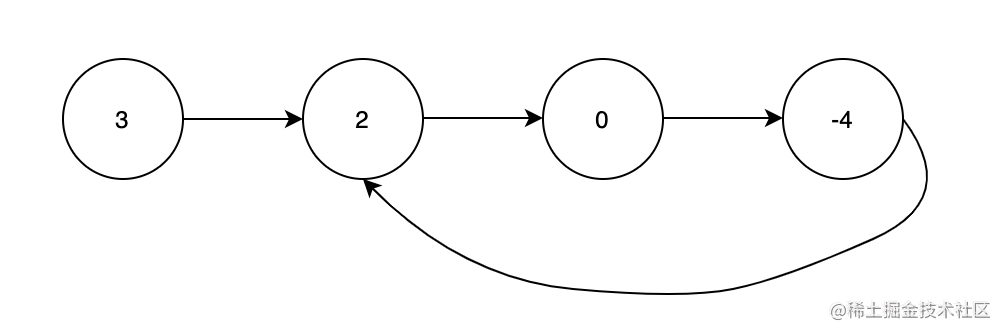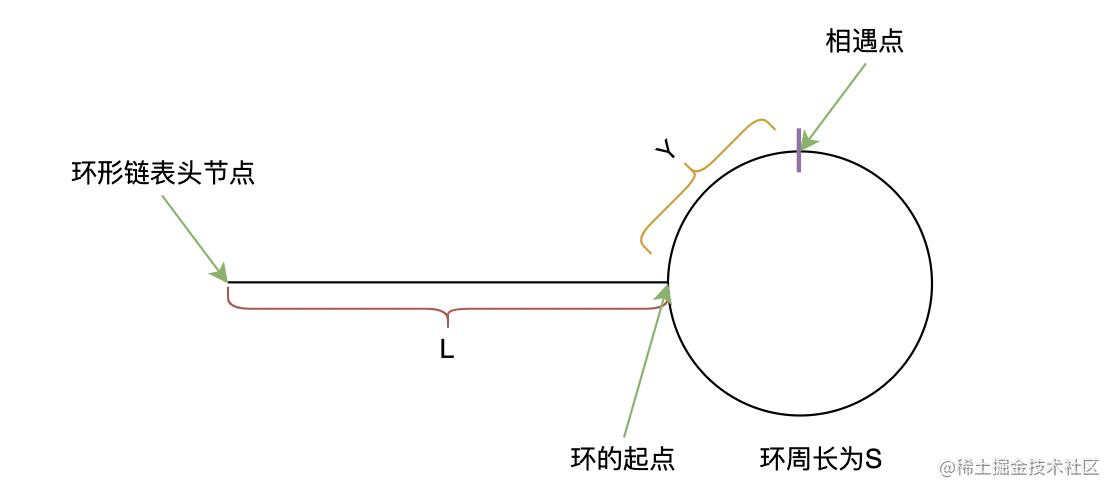# 环形链表

## 一、环形链表

### 题目输入：head = [3,2,0,-4], pos = 1

### 思路

1. 先初始化快慢指针 fastslow 指向头节点
2. 快指针每次走两步，慢指针每次走一步
3. 若链表成环，最终快慢指针会指向同一个节点
4. 若链表不成环，快指针会先走到链表的尾部

### 代码

function hasCycle(head) {
// 初始化快慢指针
let fast = slow =  head;
while(fast !== null && fast.next !== null){// 链表可能有奇数个节点或偶数个节点
// 慢指针每次走一步
slow = slow.next;
// 快指针每次走两步
fast = fast.next.next;
// 如果成环必相遇
if(slow === fast) return true;
}
// 快指针走到链表尾时还没相遇，说明不成环
return false;
};

## 二、环形链表II

### 题目输入：head = [3,2,0,-4], pos = 1

### 思路

👭友情提示：下面的证明会牵涉到一些数学运算，可能有点绕，但是认真阅读、仔细思考后应该都能看懂

$证明如下 \Downarrow$$2x=m*S+Y+L \text{ (1)}$

$x=n*S+Y+L \text{ (2)}$

$(2)*2-(1)$ 可得 $0=(2n-m)*S+Y+L$ ，即 $L=(m-2n)*S-Y$。由于 $L\geqslant 0$ ，所以 $m-2n$ 的值应为 $\geqslant 1$ 的整数，那么可得 $L=(m-2n-1)*S+S-Y$。不妨令 $p=m-2n-1$，此时 $L=p*S+S-Y$，其中 $p\geqslant 0$

$证明 \quad 毕 \Uparrow$

### 代码

// 若一个链表成环，返回环的起始节点
// 初始化快、慢指针指向头节点
while (fast !== null && fast.next !== null) {
// 慢指针每次前进一步
slow = slow.next;
// 快指针每次前进两步
fast = fast.next.next;
// 如果存在环，快慢指针必然相遇，退出当前while循环
if (slow === fast) break;
}
// 让快指针重新指向头节点
while(true){
// 让快慢指针以相同步伐前进
slow = slow.next;
fast = fast.next;
// 快慢指针相遇的点即为环的起始节点
if(slow === fast) return slow;
}
}

• 第一：如何想到利用快慢指针去解这种环形链表的题呢？
• 多刷题呗
• 第二：如何证明快慢指针的思路是正确的呢？
• 需要一定的数学推理能力